## 2.01.2012

### Pascal Triangle

Q. Write a C program to generate pascal triangle.
OR
Q. Write a C program to print following number triangle :
 1 1 1 1 2 1 1 3 3 1 1 4 6 4 1

Ans.

How to build Pascal triangle:

### =  1 , 4 , 6 , 4 , 1

/*c program for making pascal triangle*/
#include<stdio.h>
#include<conio.h>
long calc( int );
int main()
{
int i,j,row,pas;
printf("Enter no. of rows in pascal triangle : ");
scanf("%d", &row);
for(i=0; i<row; i++)
{
for(j=0; j<=(row-i-1); j++)
printf(" ");
for(j=0; j<=i; j++)
{
pas=calc(i)/(calc(j)*calc(i-j));
printf("%ld ",pas); //take single space
}
printf("\n");
}
getch();
return 0;
}

long calc( int num)
{
int x;
long res=1;
for(x=1; x<=num; x++)
res=res*x;
return (res);
}

Output:-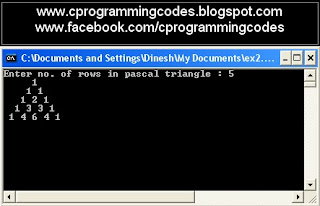Figure: Screen shot for pascal triangle C program

#### 37 comments:

1.simple way with out using function....

#include
#include

void main()
{
int i,j,n,c,k,space;
clrscr();
printf("Enter the limit ");
scanf("%d",&n);
printf("\n\n");
space=n;
for(i=0;i<=n;i++)
{
c=1;
for(k=space;k>=0;k--)
printf(" ");

space--;
for(j=0;j<=i;j++)
{
printf("%d ",c);
c=(c*(i-j)/(j+1));
}
printf("\n");
}
getch();
}

1.good its so simple, thanks

2.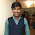Excellent ... and great effort... U proved u r a great programmer :)

3.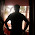it works good but if u enter the no of rows as 2,in the output three rows are printed i.e. if u enter the no rows as a,the output is printed with a+1 rows

4.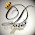@Aravind Swamy,

I checked above program again and find that,
the output will be equal of the entered no of rows.

Try some different compiler.

5.thank u dinilps
this program is working

6.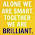friend i want to algorith

7.i dint undestand what u ment by initializing i and j kindly assist am a new lleaner

8.For those who are getting (n+1)th row as output

Try this code...

#include

void main()
{
int i,j,n,c,k,space,q;

printf("Enter the limit ");
scanf("%d",&n);
printf("\n\n");

space=n;
for(i=0;i<=n;i++)
{
c=1;
for(k=space;k>=0;k--)
printf(" ");
q=i-1;

space--;
for(j=0;j<=q;j++)
{
printf("%d ",c);
c=(c*(q-j)/(j+1));
}
printf("\n");
}

9.not working for limit grater then 5. this is not universal, using upto 4. and also there is bug of space, there is 3 rows are blank and after that printing started

10.this is not working more than 5 rows. and also 3 or 4 rows are blank and then printing starts

2.i think the comment is the best method to solve it.the given is quite logical bt the next is ultimate.

3.the above prog is good bt u hv to declare the pass as long else it give u garbage value...

4.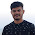Simpler Approach!
#include
void main()
{
int i,j,x,n,s;
printf("Enter The Number : ");
scanf("%d",&n);
for(i=0;i<=n;i++)
{
x=1;
for(s=1;s<=n-i;s++)
printf("\t");
for(j=1;j<=i+1;j++)
{
printf("%d\t\t",x);
x=x*(i-j+1)/j;
}
printf("\n");
}
}

1.every thing is correct except that in:
line 11 : printf(" ");
line 14 : printf("%d ",x); //single space

change these things in the program and it will run

2.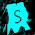Right jai raj

5.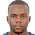its not printing the required output

1.@artwel junior,

Write down your code, for solving error.

Try some other differ compiler.

6.#include
#include
void main()
{
int i,j,sp,num=1,r;
clrscr();
for(i=1;i<=5;i++)
{
r=num;
for(sp=i;sp<=5;sp++)
printf(" "); //2 space
for(j=1;j<=i;j++)
{
printf("%d ",r%10); //3 space
r=r/10;
}
num=num*11;
printf("\n");
}
getch();
}

1.not working in quincy ...

7.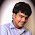can i have a flow chart and algorithm for this?

8.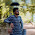This comment has been removed by the author.

9.This comment has been removed by a blog administrator.

10.can any one explain this logic for me

11.12.13.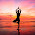#include
#include

void main()
{
int i,j,n,c,k,space;
clrscr();
printf("Enter the limit ");
scanf("%d",&n);
printf("\n\n");
space=n;
for(i=0;i<=n;i++)
{
c=1;
for(k=space;k>=0;k--)
printf(" ");

space--;
for(j=0;j<=i;j++)
{
printf("%d ",c);
c=(c*(i-j)/(j+1));
}
printf("\n");
}
getch();
}

14.#include
#include

void main()
{
int i,j,n,c,k,space;
clrscr();
printf("Enter the limit ");
scanf("%d",&n);
printf("\n\n");
space=n;
for(i=0;i<=n;i++)
{
c=1;
for(k=space;k>=0;k--)
printf(" ");

space--;
for(j=0;j<=i;j++)
{
printf("%d ",c);
c=(c*(i-j)/(j+1));
}
printf("\n");
}
getch();
}

15.#include
#include
long calc( int );
int main()
{
int i,j,row,pas;
printf("Enter no. of rows in pascal triangle : ");
scanf("%d", &row);
for(i=0; i<row; i++)
{
for(j=0; j<=(row-i-1); j++)
printf(" ");
for(j=0; j<=i; j++)
{
pas=calc(i)/(calc(j)*calc(i-j));
printf("%ld ",pas); //take single space
}
printf("\n");
}
getch()

16.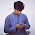#include
#include
#include

int fact(int a)
{
int b,fact=1;
for(b=1;b<=a;b++)
fact=fact*b;

return fact;

}

main()
{
int a,b,c,row,ele;
printf("Enter number of row you want to print:");
scanf("%d",&row);
for(a=0;a<=row;a++)
{
for(b=0;b<=(row-a+2);b++)
{
printf(" ");

}
for(c=0;c<=a;c++)
{
ele=fact(a)/(fact(c)*fact(a-c));
printf("%d ",ele);
}
printf("\n");

}

}

1.functiopn is best method for pascal triangle as it uses a concept of bionomial coeff

2.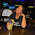am trying to run this above in my machine and is not working what is the mistake am i doing ??
can this work ??

#include
#include

int main()
{
int i,j,n,c,k,space;

printf("Enter the number to limit up on");
scanf("%d",&n);
printf("\n\n");

for(i=0;i<=n;i++)
{
c=1;
for(k=n;k>=0;k--)
printf(" ");

n--;
for(j=0;j<=i;j++)
{
printf("%d ",c);
c=(c*(i-j)/(j+1));
}
printf("\n");
}
return 0;
}

17.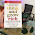54381
4381
381
81
1
aisa parogram kise ko ata ha yaha

1.#include
#include
void main()
{
int i,j,a={5,4,3,8,1};
for(i=5;i>0;i--)
{
for(j=1;j<5;j++)
{
printf("%d",&a[j]);
}
printf("\n");
}
getch();
}

18.void main()
{
int i,j,n,c,k;

printf("Enter the limit ");
scanf("%d",&n);
printf("\n\n");
for(i=0;i<=n;i++)
{
c=1;
for(k=0; k<=n-i; k++)
{
printf(" ");
}
for(j=0; j<=i; j++)
{
printf("%d ",c);
c=(c*(i-j)/(j+1));
}
printf("\n");
}
getch();
}

19.here error is not present but op is blank screen

20./* NO FORMATTED
With O(n^2) time complexity and O(n) space complexity
Able to print up to 68 rows before it overflows
/* Take less than 1ms (without output)

void pt(unsigned nRows) {
if (nRows) {
unsigned long long *prevRow = NULL, *currRow;
unsigned r,i;
printf("1\n");
for (r = 2; r <= nRows; r++) {
currRow = (unsigned long long*)malloc(r * sizeof(unsigned long long));
currRow = 1; currRow[r-1] = 1;
printf("1\t");
for (i = 1; i < r-1; i++) {
printf("%llu\t", currRow[i] = prevRow[i-1] + prevRow[i]);
}
printf("1\n");
free(prevRow);
prevRow = currRow;
}
free(prevRow);
}
}

int main() {
pt(68u);
return 0;
}